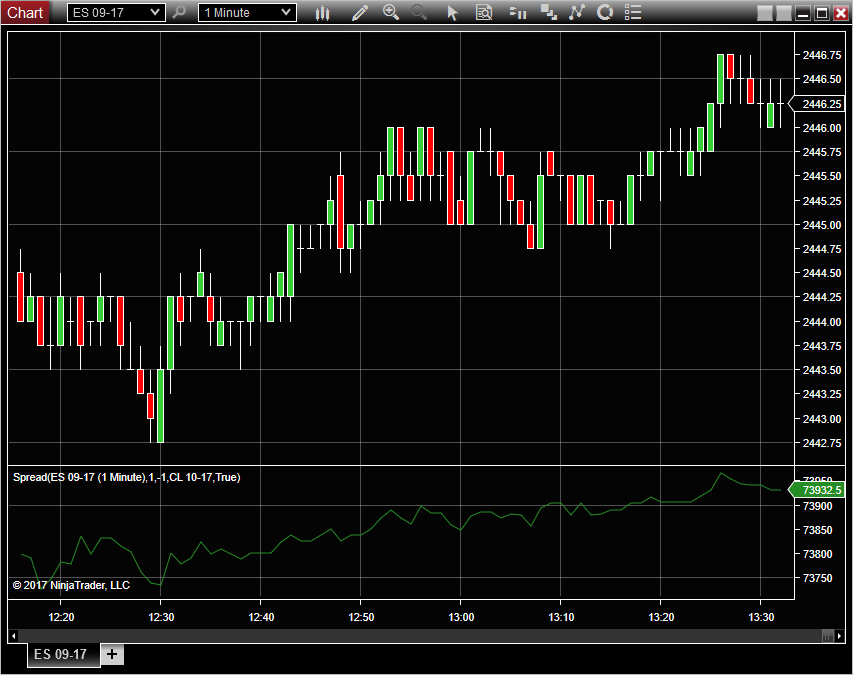Plots the Spread of two instruments

The first instrument is the primary chart data series. The secondary instrument is specified by the parameter "Symbol2".

The Parameters "Qty1" and "Qty2" are multipliers which are applied to each instrument's price before adding the two; default values are "Qty1=1" and "Qty2=‐1" which will simply plot the difference between the two instruments (P1‐P2). They may be set to other values, for example in pairs trading where the quantities might be set for roughly equal dollar amounts for each instrument. Also useful with Interactive Brokers' "Generic Combo" orders.

The Spread indicator may be used as the input to other indicators such as Bollinger Bands, to plot its relationship to its recent volatility.

The "UseMultiplier" parameter defaults to "true" to incorporate the contract multiplier into the calculation.

Default behavior is "Calculate.OnEachTick" for real‐time display; set to "Calculate.OnBarClose" if not needed.